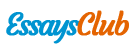# Problem Bonds

Autor:   •  August 11, 2017  •  1,636 Words (7 Pages)  •  201 Views

Page 1 of 7

...

[pic 19]

The annualized holding period yield for the first bond is 13.26%.

- Assuming the face value of the 7-year bond is \$100

The present value of this bond is

[pic 20]

[pic 21]

The future value in year 3 is

[pic 22]

Therefore,

[pic 23]

Problem 5

- The discount factors can be calculated below.

[pic 24]

[pic 25]

[pic 26]

[pic 27]

- Calculated the implied interest rate

[pic 28]

[pic 29]

[pic 30]

To prevent arbitrage, [pic 31]

Therefore,

[pic 32]

[pic 33]

[pic 34]

- Since the price of the forward rate agreement being offered by the bank is greater than the forward price determined by no-arbitrage, a arbitrage opportunity exists and I want to construct a strategy that allows me to buy the forward contract at present at the lower price and sell at the higher price in the future.

Problem 6

Solution:

As LPH holds, we assume that investors have an investment horizon of 3 years, also assume the yield curve below. Supposing the liquidity premium is 0.1%

MATURITY

YTM

1

3.5%p.a

2

3.7%p.a

3

4%p.a

4

4.1%p.a

5

4.4%p.a

- Under LPH

Strategy 1: Invest \$1 at beginning for 3 years

Since is not high enough, the initial yield is high enough to make investors earn a reasonable return.[pic 35]

[pic 36]

Strategy 2: Invest \$1 at beginning for 2 years and reinvest in year 2 for another 1 year.

[pic 37]

[pic 38]

[pic 39]

Strategy 3: Invest \$1 at beginning for 5 years and sell it in year 3

[pic 40]

[pic 41]

Let be the expected value of \$1 at , [pic 42][pic 43]

Then,

[pic 44]

- UNDER PEH

Strategy 1: Invest \$1 at beginning for 3 years

The forward rate has be locked in as the event happen.

[pic 45]

Strategy 2: Invest \$1 at beginning for 2 years and reinvest in year 2 for another 1 year.

[pic 46]

[pic 47]

[pic 48]

Strategy 3: Invest \$1 at beginning for 5 years and sell it in year 3

[pic 49]

[pic 50]

Let be the expected value of \$1 at , [pic 51][pic 52]

Then,

[pic 53]

Based on the calculation above, we can get a graph below. From the graph, we can see that the vertical axis represent the expected returns and the horizon axis represent three different strategies. We can clearly conclude that under PEH the expected return is a flat curve. However, the LPH is a downward-sloping curve. As to say to strategy 3 the curve become upwards, it is because the liquidity of the bond for a long time bond is very risk. The investors will not change their mind unless is high enough. Therefore, the expected return is higher than its usual condition.[pic 54]

[pic 55]

Problem 7

Solution:

As PHT holds, we assume that investors have an investment horizon of 3 years, also assume the yield curve below.

MATURITY

YTM

1

3.5%p.a

2

3.7%p.a

3

4%p.a

4

4.1%p.a

5

4.4%p.a

Normally, under PEH, every strategy has the same forward rate. Investors do not have preferred habitat.

We suppose that

[pic 56]

- A positive risk premium happens under PHH, when investors have much more demand for 2 years bond. In other words, investors have a preferred habitat to buy 2-year bonds.[pic 57]

A new 2-year YTM =4.4%

A new 3-year YTM =4.5%

[pic

...